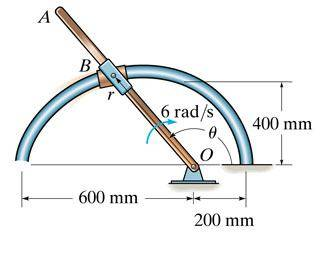# Circular Motion Kinematics

## Homework Statement

The rod OA rotates clockwise with a constant angular velocity of 6 rad/s. Two pin-connected slider blocks located at B move freely on OA and the curved rod whose shape is a limacon described by the equation r = 200*(2-cosθ) mm. Determine the magnitude of the acceleration of the slider blocks at the instant θ = 135°.αr=r''-rθ'2
αθ=rθ''+2r'θ'
α = √(αr2θ2)

## The Attempt at a Solution

I am trying to solve for the magnitude using the normal and tangential acceleration components.
r = 200*(2-cosθ) --> r(135) = 541.42 mm θ' = 6 rad/s
r' = 200 sinθ * θ' --> r'(135) = 848.53 mm/s θ'' = 0
r'' = 200cosθ * θ'2= -5091.17 mm/s2

When I plug all those values in I keep getting the wrong answer. Can someone tell me where I am going wrong?

TSny
Homework Helper
Gold Member
Careful with the signs. Note that the rod is rotating clockwise.

Careful with the signs. Note that the rod is rotating clockwise.

Can you explain that more? Is this because there is a negative change in θ over time?

I ended up getting the right answer, must have just been a miscalculation.

TSny
Homework Helper
Gold Member
Can you explain that more? Is this because there is a negative change in θ over time?
Yes. In the figure, ##\theta## increases in the counterclockwise direction. Therefore, if the rod rotates clockwise, ##\dot{\theta}## is a negative number. You should find that ##\dot{r}## is negative when ##\theta = 135^o##.

Yes. In the figure, ##\theta## increases in the counterclockwise direction. Therefore, if the rod rotates clockwise, ##\dot{\theta}## is a negative number. You should find that ##\dot{r}## is negative when ##\theta = 135^o##.

Okay I understand that. Thank you for explaining. I just go lucky in my calculations because they ended up cancelling the negatives out.